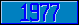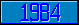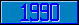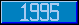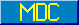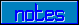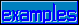## JIS X0208–1990

### 3. JIS X0208–1990

In JIS X0208–1990, the relation of decimal and character is obtained as following. Let C1 and C2 be the decimal values of the 1st byte and the 2nd byte code for character, then the range of decimal code for both C1 and C2 is [33,127] and the decimal value of the character is C1*256+C2. Let n be a decimal and if there is no character assigned for n in JIS X0208, then \$C(n) is a space as exemplified by \$C(8481).